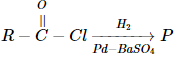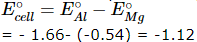Courses

# AFMC Chemistry Mock Test - 4

## 50 Questions MCQ Test AFMC Mock Test Series 2021 | AFMC Chemistry Mock Test - 4

Description
This mock test of AFMC Chemistry Mock Test - 4 for NEET helps you for every NEET entrance exam. This contains 50 Multiple Choice Questions for NEET AFMC Chemistry Mock Test - 4 (mcq) to study with solutions a complete question bank. The solved questions answers in this AFMC Chemistry Mock Test - 4 quiz give you a good mix of easy questions and tough questions. NEET students definitely take this AFMC Chemistry Mock Test - 4 exercise for a better result in the exam. You can find other AFMC Chemistry Mock Test - 4 extra questions, long questions & short questions for NEET on EduRev as well by searching above.
QUESTION: 1

Solution:
QUESTION: 2

Solution:
QUESTION: 3

### In the following reaction ,product P isSolution:
QUESTION: 4

The total number of possible values of magnetic quantum number for the value of l = 3 is

Solution:
QUESTION: 5

The quantum number values for the designation 3d are

Solution:
QUESTION: 6

ACTH is secreted by

Solution:
QUESTION: 7

Vanaspati ghee is prepared by which of the following reactions of vegetable oils?

Solution:
QUESTION: 8

The fatty acid which shows reducing property is

Solution:
QUESTION: 9

The molecule having zero dipole moment is

Solution:
QUESTION: 10

The oxidation of SO₂ by O₂ to SO₃ is an exothermic reaction. The yield of SO₃ will be maximum if

Solution:
QUESTION: 11

The reaction N₂ + O₂ → 2NO is endothermic . The forward reaction is

Solution:
QUESTION: 12

An endothermic reaction A → B has an activation energy 15 kcal/mole and energy of reaction 5 kcal/mole. The activation energy of the reaction B → A is

Solution:
QUESTION: 13

A first order reaction is 10% complete in 20 min. The time taken for 19% completion is

Solution:
QUESTION: 14

Electron affinity depends on

Solution:

As the size of the atom increases, the electron affinity decreases, whereas when the nuclear charge increases the electron affinity increases.

QUESTION: 15

The radii of F, F -, O and O2- are in the ordera

Solution:

As we move from left to right in a period atomic radius decreases. Moreover, as the number of electrons in anion increases, the effective nuclear charge and attractive force on the outer electrons decreases. Thus, the anion with highest charge always possess largest ionic radius.

QUESTION: 16

Which of the following is an antibiotic?

Solution:
QUESTION: 17

Which of the following holds good to the laws of thermodynamics for the reaction C₂H₄(g) + 3O₂(g) → 2CO₂(g) + 2H₂O(l)

Solution:
QUESTION: 18

Medicine which is an antibiotic is

Solution:
QUESTION: 19

The cell reaction of the cell is Mg(s) + Cu+2 (aq) → Cu(s) + Mg+2 (aq). If the standard reduction potential of magnesium and copper are -2.37 V and +0.34V respectively, the standard EMF of the cell is

Solution:
QUESTION: 20

The arrangement of hydrogen atoms around carbon in the methane molecule is

Solution:
QUESTION: 21

The e.m.f. of a galvanic cell with electrode potentials of Al equal to -1.66 V and that of Mg equal to - 0.54 V is

Solution:

E.M.F. can be positive only if oxidation takes place at Mg-electrode. But here the reduction reaction takes place.QUESTION: 22

The ionic mobility of alkali metal ions in aqueous solution is maximum for

Solution:
QUESTION: 23

Strongest bond is in between

Solution:
QUESTION: 24

Main process for the manufacture of sodium carbonate is

Solution:
QUESTION: 25

Which one of the following substances is used in the laboratory for a fast drying of neutral gases?

Solution:
QUESTION: 26

Benzene on treatment with a mixture of conc.HNO₃ and conc. H₂SO₄ at 100°C gives

Solution:
QUESTION: 27

When acetylene is passed through dil. H₂SO₄ in the presence of HgSO₄, the compound formed is

Solution:
QUESTION: 28

In which of the following acid-base titration, pH is greater at eqivalence point?

Solution:
QUESTION: 29

H₂O₂ is commonly prepared in lab. by the reaction of

Solution:
QUESTION: 30

When a slow neutron goes sufficently close to a U-235 nucleus, then the process which takes place is called

Solution:
QUESTION: 31

A radioactive nucleus emits a β-particle. The parent and the daughter nuclei are

Solution:
QUESTION: 32

The half-life of a radioactive isotope is 2.3 hours. Its disintegration constant will be

Solution:
QUESTION: 33

Wet ether is not used as a solvent in Wurtz reaction, because the water present in it

Solution:
QUESTION: 34

Which of the following will exhibit tautomerism?

Solution:
QUESTION: 35

Which of the following is least basic?

Solution:
QUESTION: 36

Nitrogen is relatively inactive element because

Solution:
QUESTION: 37

Boric acid is polymeric due to

Solution:
QUESTION: 38

Which would quickly absorb oxygen?

Solution:
QUESTION: 39

When lightning flash is produced through air, the gas formed is

Solution:
QUESTION: 40

Nylon-6,10 is a condensation polymer of hexamethylenediamine and

Solution:
QUESTION: 41

Which is not a macromolecule?

Solution:
QUESTION: 42

When iron is added to CuSO₄ solution, copper is precipitated. It is due to

Solution:
QUESTION: 43

Water is a/an

Solution:
QUESTION: 44

Azeotropic mixtures are

Solution:
QUESTION: 45

The temperature at which real gases obey the ideal gas laws over a wide range of pressure is called

Solution:
QUESTION: 46

An ideal gas cannot be liquefied because

Solution:
QUESTION: 47

Brownian movement is found in

Solution:
QUESTION: 48

Indicate the mineral from which copper is manufactured

Solution:
QUESTION: 49

Bell metal is an alloy of

Solution:
QUESTION: 50

Near the top of the blast furnace used for the extraction of iron, the metal oxides are reduced to spongy iron by

Solution: